New update is available. Click here to update.

# Tournament Tree (Winner Tree) and Binary Heap

## Introduction

Hey Ninjas, today we will learn about the tournament tree and binary heap. Both data structures are structured similarly, with nodes grouped in a tree-like layout. They differ, however, in how they store and retrieve data, which affects their performance and efficiency in various use scenarios.

In the upcoming sections, we will look at the essentials of Tournament Trees.

## What is a Tournament Tree?

The tournament tree is an application of the heap data structure. It is a binary heap; hence the tournament tree is also a complete binary tree and satisfies the heap-order property.

As the name suggests, a tournament tree comes from the idea of a tournament or a competition. If there are p players in a tournament, then the tournament tree will have p-leaf nodes which can also be called external nodes, and it’ll have p-1 internal nodes. The tournament trees are also known as selection trees.

Add some important properties of tournament trees in bulleted form below

Also see, Implementation of Heap.

## Types of tournament trees

1. Winner Trees
2. Looser Trees

### Winner Trees

The idea here is that in a winner tree, the nodes at a particular level store the winner of the two nodes at the level below it. Hence, the tree’s root node stores the overall winner of the competition.

For instance, if we consider that in a tournament there are a total of eight players (1, 2, 3, 4, 5, 6, 7, 8) and in a match between any two players among these, the player with numerically smaller value wins the match (minimum winner tree).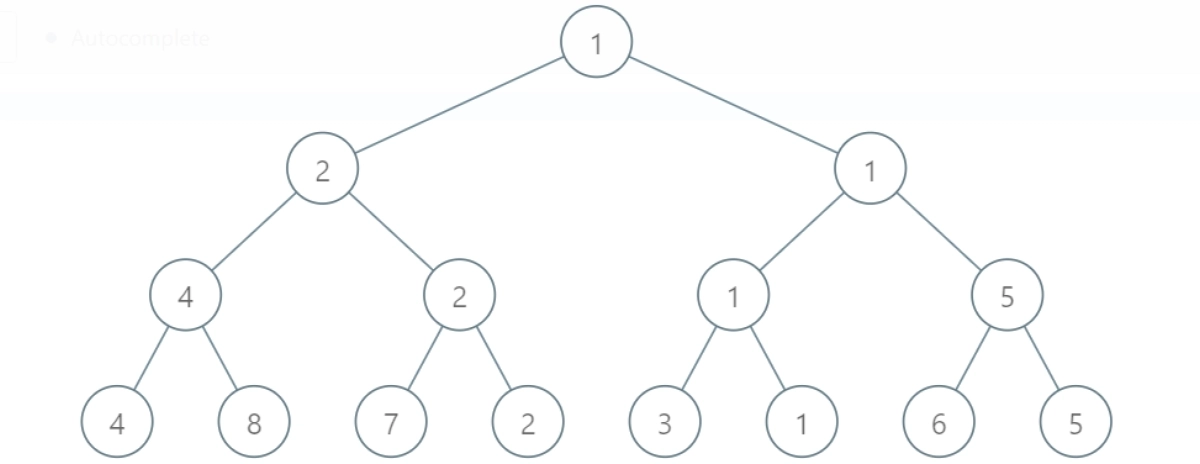The winner tournament tree constructed for the above example will look like this.

Clearly, 1 is the winner here because it is the numerically smallest element in the group.

If we observe the tree, we’ll see that it is a min-heap because, along with being a complete binary tree, the value of each node is lesser than or equal to the value of its children.

Note: A tournament tree will always be a heap, but a heap is not always a tournament tree because, in a tournament tree, the value of each node must be the value of its left or right child, but in a heap, a node can have any value lesser than it’s left and right child.

### Looser Trees

The loser tree is another type of tournament tree in which the nodes at a particular level store the loser of the two nodes at the level below it. In a Looser tree, the tournament’s winner is stored at the top, and the second place (runner-up) is the child of it.

So, if we are considering a numerically smaller value as the winner, then each node’s value in a Looser Tree will be the greater value among both of its children.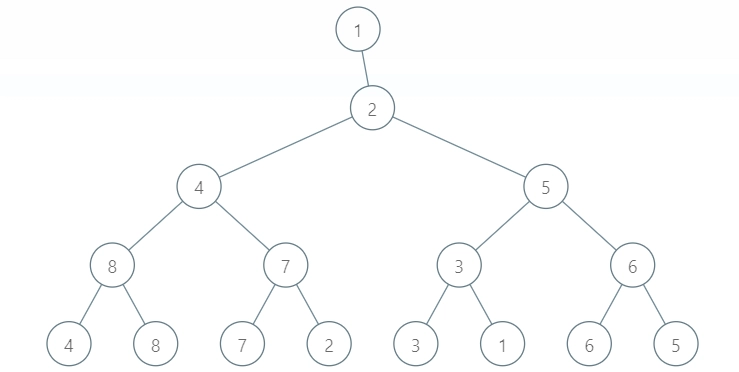The loser tree constructed for our example will look like this.

One advantage of looser trees compared to winner trees is that it is sufficient to examine the nodes on the path from leaf nodes to the root node for restructuring the tree in looser trees.

For each of the matches, the time complexity is O(1), and in total, there are n-1 nodes (internal nodes); hence the time complexity to initialize the tree is O(n).

## Finding Median of the Sorted Arrays (Tournament Trees Approach)

One of the Tournament Trees applications is that we can find the median of N sorted arrays. For instance, if we have N ascending order sorted arrays, we can efficiently use minimum winner tournament trees to find the median.

The arrays will act as players (leaf nodes of the tournament tree), and the winner element from respective arrays will occupy the internal nodes.

### Demonstration

Assume that we have 3 sorted arrays of different sizes:

Array 1 = {2, 5, 7, 11, 15},

Array 2 = {1, 3, 4}, and

Array 3 = {6, 8, 12, 13, 14}

The respective sizes of arrays are 5, 3, and 5; hence the median will be the 7th element of the overall array.

Sorted merged array = {1, 2, 3, 4, 5, 6, 7, 8, 11, 12, 13, 14, 15}

Median = 7th element = 7

Let’s solve this using winner tournament trees.

Initially, the leaf node will contain the first element of the respective sorted arrays. Since there are three arrays, we’ll assume that the fourth leaf node is either null or contains some very large value (9999999).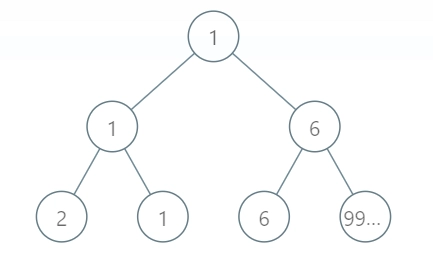Here 1 is the winner, so we replace the leaf node with the next value from the respective array. (i.e., node 3 will replace node 1)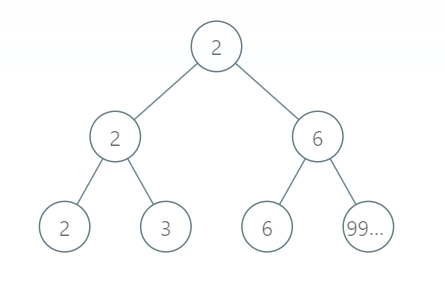Now, 2 is replaced by 5. These steps will continue till we get the 7th element from the tournament tree.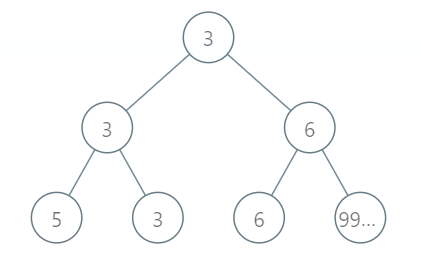Now since array 2 is empty, 4 will be replaced with a very large value 9999999.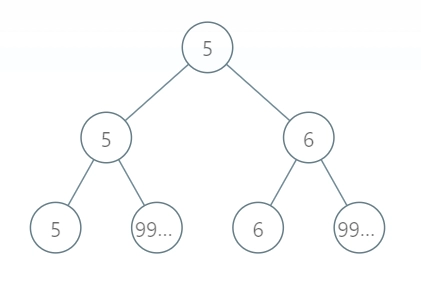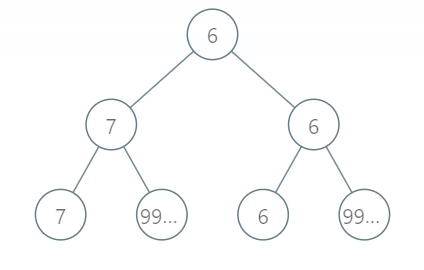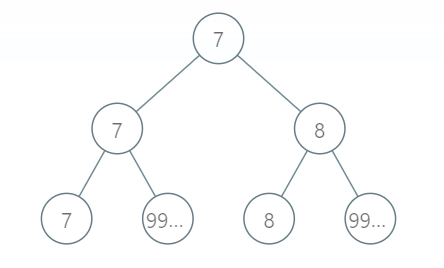Finally, after the 7th iteration, we will get our median which is 7.

The time required to merge N sorted arrays of size M (assuming all arrays have the same size) is O(M*logN), and the time required to find the median will be O(m*logN), m being the median position (7 in our above example).

## Application of Tournament Trees

Tournament Trees find their applications in a wide range of areas within computer science and programming.

• Sorting algorithms such as Tournament Sort use this data structure to efficiently sort sets of elements.
• Similarly, the scheduling of matches and tournaments in games and sports also employs tournament trees.
• In image processing, these trees find use in determining the minimum or maximum value of pixels in an image.
• Moreover, tournament trees are also useful in decision-making algorithms, where they help in selecting the best option among a set of alternatives.

### What is tournament tree in data structure in C?

A tournament tree is a binary tree that keeps track of the minimum (or maximum) element in a set of values. It is built by recursively comparing adjacent elements and creating a binary tree of the winners until only one winner is at the root.

### What are the properties of tournament tree?

The properties of a tournament tree include the following:

• It is a complete binary tree.
• The leaf nodes represent the initial elements of the set.
• The internal nodes represent the winners of the child nodes.
• The root node holds the overall winner of the set.

### What is an example of a winner tree?

One example is a set of integers (3, 7, 2, 5, 1, 8, 4, 6) where a tournament tree can be built by recursively comparing adjacent elements and creating a binary tree of the winners until there is only one winner at the root node.

### What is tournament sort in data structure?

It is a sorting algorithm that utilises a tournament tree. The algorithm first builds a tournament tree from the given set of elements and then extracts the minimum (or maximum) element from the tree's root node until all the elements are sorted.

## Conclusion

Congrats on learning something new today!!

In this blog post, we learned a new and fundamental topic: Tournament Trees. We discussed types of tournament trees and how to find the median of the sorted arrays. We also looked at the application of the tournament tree and some frequently asked questions.